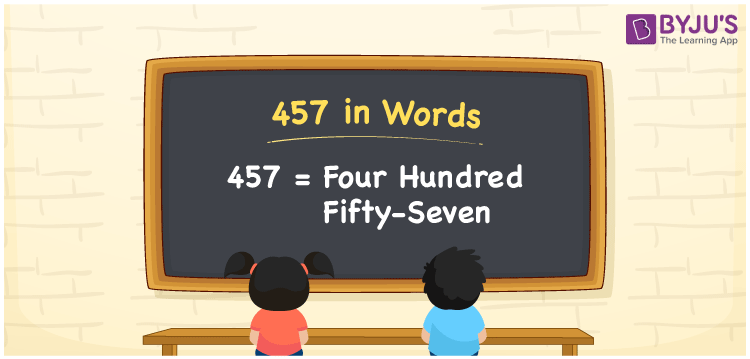# 457 in Words

457 in words is Four hundred fifty-seven. We know that the number name of 457 is Four hundred fifty-seven. Generally, we use number names to spell or express those numbers to others. For instance, you are asked to read the amount Rs. 457, then you may read it as “Four hundred fifty-seven rupees”. Let’s learn how to convert the cardinal number 457 into words here in this article.

 457 in words Four hundred fifty-seven Four hundred fifty-seven in Numbers 457

## 457 in English Words

Generally, we use the English alphabet to write numbers in words. So, we spell 457 in English words as “Four hundred fifty-seven”.## How to Write 457 in Words?

We know that 457 is a three-digit number so, let’s create a place value chart with three columns such that each digit is expressed with the respective place value. This can be done as given below.

 Hundreds Tens Ones 4 5 7

Here, ones = 7, tens = 5, hundreds = 4

The above digits can be expanded as follows.

4 × Hundred + 5 × Ten + 7 × One

= 4 × 100 + 5 × 10 + 7 × 1

= 400 + 50 + 7

= Four hundred + Fifty + Seven

= Four hundred fifty-seven

Therefore, 457 in words = Four hundred fifty-seven

As we know, 457 is a natural number that precedes 458 and succeeds 456.

457 in words – Four hundred fifty-seven

Is 457 an even number? – No

Is 457 an odd number? – Yes

Is 457 a prime number? – Yes

Is 457 a composite number? – No

Is 457 a perfect square number? – No

Is 457 a perfect cube number? – No

## Frequently Asked Questions on 457 in Words

Q1

### How do you write 457 in words?

We can write the number 457 in words as Four hundred fifty-seven.
Q2

### Write 389 + 457 in words.

389 + 457 = 846 Thus, we can write the value of 389 + 457, i.e., 846 in words as Eight hundred forty-six.
Q3

### How to write Rs. 457 in words on a cheque?

On a cheque, we generally write an amount of Rs. 457 in words as “Four hundred fifty-seven rupees only”.